All submissions of the EM system will be redirected to Online Manuscript Submission System. Authors are requested to submit articles directly to Online Manuscript Submission System of respective journal.

# Effects of Ternary Mixed Crystal And Layer Thickness on I-V Output Characteristics of Al2O3/AlGaN/GaN MOSHEMTs

Zhou XJ1, Xing Y2, Gu Z3 and Meng GQQG1*

1Department of Physics, Ordos Institute of Technology, Ordos, China

2Department of Physics, Inner Mongolia University, Hohhot, China

3Department of Mathematics and Physics, Shijiazhuang Tiedao University, Shijiazhuang, China

*Corresponding Author:
Meng GQQG
Department of Mathematics and Physics,
Shijiazhuang Tiedao University,
Shijiazhuang,
China;
Email: 497029230@qq.com

Received: 26-Apr-2023, Manuscript No. JPAP-23-97039; Editor assigned: 28-Apr-2023, Pre QC No. JPAP-23-97039 (PQ); Reviewed: 12-May-2023, QC No. JPAP-23-97039; Revised: 26-Jun-2023, Manuscript No. JPAP-23-97039 (R); Published: 03-Jul-2023, DOI: 10.4172/2320-2459.11.4.003

Citation: Meng GQQG, et al. Effects of Ternary Mixed Crystal and Layer Thickness on I-V Output Characteristics of Al2O3/AlGaN/GaN MOSHEMTs. RRJ Pure Appl Phys. 2023;11:003.

Copyright: © 2023 Meng GQQG, et al. This is an open-access article distributed under the terms of the Creative Commons Attribution License, which permits unrestricted use, distribution and reproduction in any medium, provided the original author and source are credited.

Visit for more related articles at Research & Reviews: Journal of Pure and Applied Physics

## Abstract

The threshold voltages and output currents in Al2O3/AlGaN/GaN MOSHEMTs are calculated by the charge control model under the total depletion approximation taking into account the fixed charges at the Al2O3/AlGaN interface and the polarization in each layer. The results demonstrate that the threshold voltages drift negatively as the Al component in AlGaN and the thickness of each layer rise. It has also been discovered that increasing Al component and Al2O3 layer thickness results in a significant increase in current, whereas increasing the AlGaN barrier thickness has only a minor impact. These results imply that the performance of MOSHEMT devices can be improved by the ternary mixed crystal and the size effects.

#### Keywords

MOSHEMTs; I-V output characteristics; Threshold voltage; Ternary mixed crystal effect; Size effect

#### Introduction

The fabrication of a High Electron Mobility Transistor (HEMT) usually relies on a hetero junction based on two materials with different band gaps. Because the Two-Dimensional Electron Gas (2DEG) with a high concentration exsits near the AlGaN/GaN interface, which can significantly improve the electrical performance of devices, AlGaN/GaN hetero junctions are more suitable for making HEMTs. However, the large leakage current and current collapse severely hampered the development of traditional AlGaN/GaN hetero junction transistors in recent decades. Subsequently, this limitation was alleviated since the appearance of Metal-Oxide-Semiconductor High Electron Mobility Transistors (MOS HEMTs). As a result, the properties of MOSHEMTs, such as I-V output characteristics, trans conductance and capacitance, have gotten a lot of attention .

#### Materials and Methods

Many experimental and simulation studies on MOSHEMTs were conducted in the early years of this decade. For example, an Al2O3/AlGaN/GaN MOSHEMT was made by Metal Organic Chemical Vapor Deposition (MOCVD), and the effects of temperature and cap layer on capacitance were investigated, but the drain current was not provided . Meanwhile, the transfer characteristics of this structure were investigated, and an analytical expression for the threshold voltage was given. However, the effects of Al component and AlGaN barrier thickness were overlooked. Later, the drain current, trans conductance, and capacitance of AlGaN/GaN HEMTs were investigated using Technology Computer Aided Design (TCAD), and the results obtained agreed well with the experimental data . However, the effects of Ternary Mixed Crystal (TMC) and film size were not taken into account in this work. The theoretical researches, on the other hand, are relatively scarce . In fact, theoretical researches in this field are critical to the development of MOSHEMTs because of the high cost of experimental research and complicated calculations in simulation. Rashmi, et al., used the charge control model to calculate the density of 2DEG, drain current, and threshold voltage of AlGaN/GaN HEMTs in the early year, and obtained analytical expressions for these physical quantities across the entire operating region. Later, Gangwani, et al., employed the same model to investigate the drain current, transconductance, and capacitance characteristics of AlGaN/GaN HEMTs in the strong inversion region. The results of drain current and transconductance given by Rashmi and Gangwani were compared with the experiments and found to be reliable. However, the effects of TMC and size were also ignored in their works .

In the current work, the threshold voltages and output currents in Al2O3/AlGaN/GaN MOSHEMTs are studied under the charge control model, taking into account spontaneous and piezoelectric polarization, and the influences of TMC and film size on them are analyzed in detail. Our results are consistent with the experimental data and can be used to improve the properties of MOSHEMTs (Figure 1) [6-14].

Model and theory

A schematic diagram of an Al2O3/AlGaN/GaN MOSHEMT under consideration here is shown in Figure 1. The thicknesses of the Al2O3 layer and the AlGaN barrier are designated as dox and db. The dashed line in the figure represents the 2DEG, which collects in the triangle potential well near the AlGaN/GaN interface. Due to the small number of electrons in the other higher excited states, it is reasonable to assume that only the ground state E0 and the first excited state E1 are occupied by the electrons . The concentration ns of the electron gas in the well as a function of temperature T can be expressed as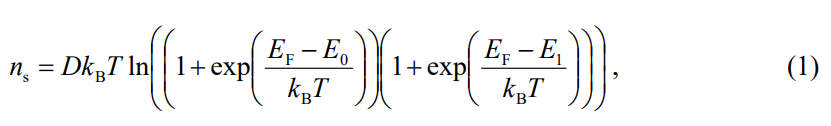Where the two dimensional conduction state density D=4πm*/h2, m* and h are the electron effective mass and the Planck constant, respectively. kB in Equation (1) is the Boltzmann constant.

EF is the fermi energy. Under the total depletion approximation, electrons diffuse from AlGaN into the triangular well and ns in Equation (1) can be obtained by solving the Poisson’s equation as follows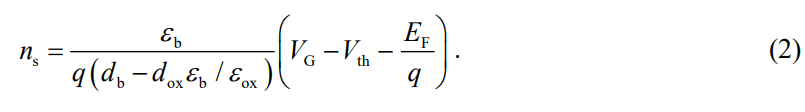Here, q is electron charge, VG is the gate bias applied. εox and εb are the relative dielectric constants of Al2O3 and AlGaN materials. Vth is the threshold voltage of the device, which can be calculated using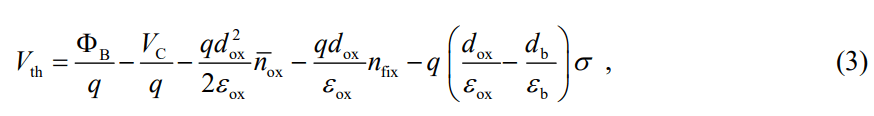where ΦB is the schottky barrier. nox is the average electron concentration in Al2O3 layer. VC is the conduction band offset between the two different materials. nfix represents the density of the fixed charges at the Al2O3/AlGaN interface. σ denotes the density of the polarization charges at the AlGaN/GaN interface. When the system is in its equilibrium state, the total charges depleted from the barrier region should be equal to the number of charges accumulated in the triangular well [16-18]. By simultaneously solving equations (1) and (2), the sheet density ns of 2DEG in weak, moderate and strong inversion regions can be obtained immediately.

Weak inversion

In this region, Fermi level EF is much lower than energy levels E0 and E1 occupied by the electrons to indicate EF<<E0, and at the same time ns is very small. As a result, Equation (1) can be approximated as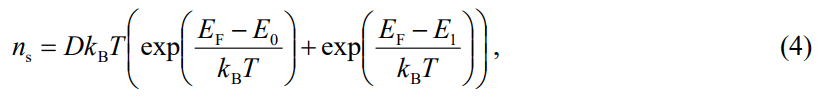and EF can be expressed as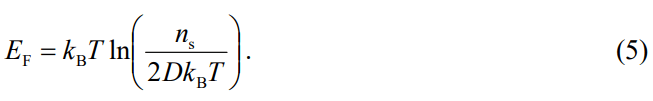Substituting equation (5) into equation (2), ns is obtained as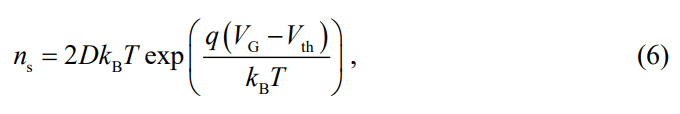then the drain current Ids can be expressed as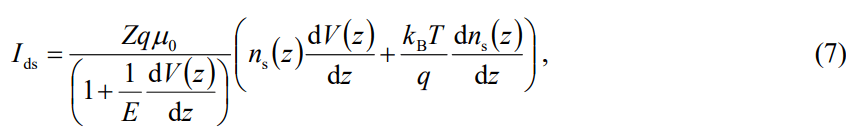where electric field E=Ecvsat/(μ0Ec-vsat) in which Ec=vsat0 is the saturated electric field, μ0 and vsat represent the low-field electron mobility and the saturation velocity, respectively. Z is the width of the gate, V(z) is the channel potential at position z. The gate voltage VG in equation (6) should be replaced with position dependent gate voltage VG-V(z), and Ids can be obtained by substituting equation (6) into equation (7), after an integral in the range of the channel length L, Ids can be written as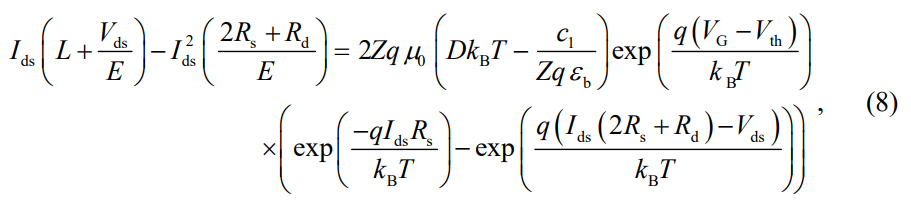where c1=ZqDkBb. The boundary conditions using in the integral are as follows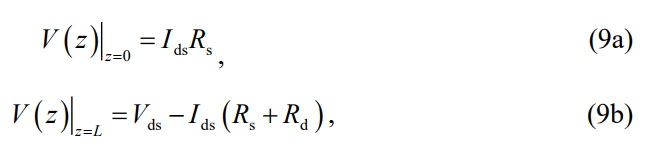Where Rd and Rs are the drain and source parasitic resistances, and Vds is the applied drain voltage.

Moderate inversion

In this region, the Fermi level is located at the bottom of the potential well, which is only a few kBT higher than the conduction band edge, so that equation (1) can be written as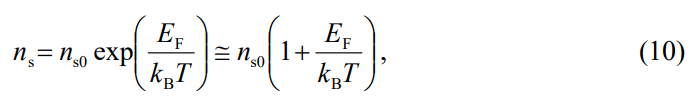where ns0 is the electron sheet density when EF=0. Instituting ns into equation (2), Fermi energy level EF in a moderate inversion mechanism can be obtained, and the electron sheet density ns can be written as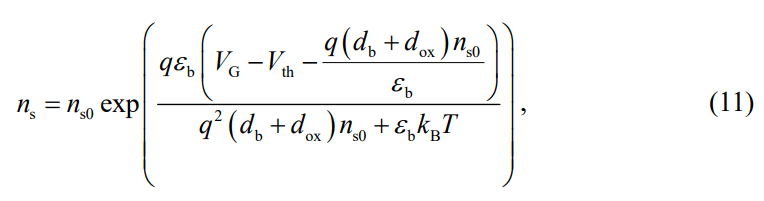Instituting equation (11) into equation (7), after an integral, the drain current Ids can be obtained as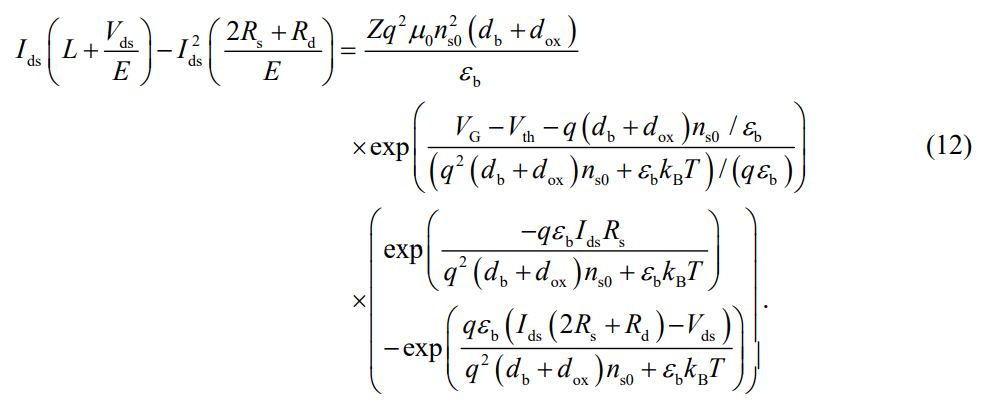Strong inversion

The working voltage of the device in this region is higher than the threshold voltage, and EF>>Ei. So that Equation (1) can be written as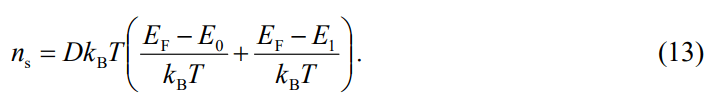Because ns is very large in the strong inversion region, Fermi energy level EF can be reduced as ns/2D

Therefore, the electron sheet density ns can be obtained as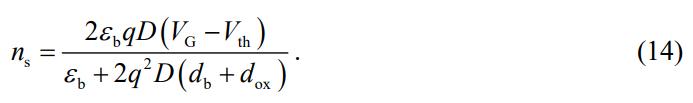Instituting equation (14) into equation (7), Ids is obtained as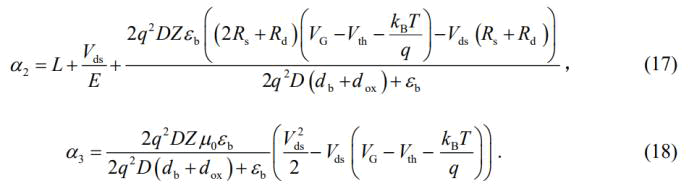#### Results and Discussion

According to Equation (3), the threshold voltages Vth are obtained in some Al2O3/AlGaN/GaN MOSHEMTs using the parameters provided by Table 1, and the calculated results are displayed in Figure 2. It can be seen that the values of Vth are negative, demonstrating that these MOSHEMTs are depletion-mode devices. The figure also demonstrates that Vth declines obviously when db (x=0.3, dox=10 nm), dox (x=0.3, db=30 nm) and x (db=30 nm, dox=10 nm) increase. The variations of Vth with dox and db are consist with the conclusion given by Tapajna, Hu. However, the influence of Al component was not studied in these references (Table 1 and Figure 2).

 Schottky barrier (eV) ΦB 2.9 Relative dielectric constants (e0 ) eb 9.5-0.5x eox 9.1 Fixed charge density (cm-2) nfix 7.08×1012 Average electron concentration (cm-3) nox 1.1×1018 Saturation velocity (cm/s) vsat 7.8 × 106 Low-field electron mobility (cm2V-1s-1) μ0 1200 Parasitic source resistance (Ω) Rs 5.8 Parasitic drain resistance (Ω) Rd 16

Table 1. Parameters used in computation.

Figure 2: Threshold voltage vs Al component x and AlGaN (Al2O3) thickness db (dox) for Al2O3/AlGaN/GaN MOSHEMTs.

For MOSHEMT devices, adding the thicknesses of Al2O3 and AlGaN layers will significantly weaken the gate electric field, which will reduce the control ability of gate voltage on the device . As a result, a higher gate voltage will be required to turn the device on or off. Similar to this, increasing the Al component will increase the dielectric constant of AlGaN material. This means that more polarized charges can be generated under the same gate voltage, and the polarized electric field's ability to counteract the gate electric field is strengthened, which also reduces the gate voltage's controllability [20-25]. Finally, Vth has a negative drift as shown in Figure 3.

Figure 3: I-V characteristics in Al2O3/AlGaN/GaN MOSHEMTs for (a) VG= -3~4V, db=25 nm, dox=20 nm, x=0.25; (b) VG=0V, db=30 nm, dox=16 nm, x=0.1~0.4; (c) VG=3V, db=10~25 nm, dox=2.4 nm, x=0.27; and (d) VG=2V, db=30 nm, dox=2~14 nm, x=0.1. Dotted lines represent the experimental values.

Figure 3 shows the I-V output characteristics of some Al2O3/AlGaN/GaN MOSHEMTs. For db=25 nm, dox=20 nm and x=0.25, the drain currents Ids under different gate voltages are given in Figure 3(a). It can be found that Ids=0 when VG=-3V, indicating the device is in the off state. At this time, the gate voltage is referred to as the cut-off voltage or the threshold voltage. When the gate voltage is higher than the threshold voltage, electrons begin to congregate near the AlGaN/GaN interface, a channel forms at the same time, and the device is turned on. As the gate voltage is gradually increased, more charges are induced in the channel inversion layer, the channel conductance rises, and the on resistance falls. As a result, the corresponding drains current Ids and saturation current go up with the increment of the gate voltage. At VG=4V, the saturation current can reach 780 mA/mm which is fairly consistent with the experimental result, as shown by the dotted line in the Figure 3(a) .

The TMC effect of Al component modulation on Ids is depicted in Figure 3(b). The figure shows that as the Al component increases, both the drain and saturation currents rise obviously. The maximum output current increases from 105 to 184 mA/mm when x changes from 0.1 to 0.4. The reason for this result is that as the Al component in AlGaN increases, the band offsets between AlGaN and GaN will increase, a deeper potential well near the interface is formed to create a stronger binding force on the electrons, resulting in more electrons that can be driven by the drain voltage to generate a larger drain current. In this case, a higher reverse voltage is required in order to turn the device off, which is consistent with the conclusion shown in Figure 2 .

Figures 3(c) and 3(d) display the size effects on Ids. The figures show that there is little difference between the curves of Ids in the variable resistance region where Vds is low. This is because the channel conductance is primarily determined by the inversion layer charge density which is regulated by an overdrive gate voltage greater than the threshold value. When Vds is increased to a certain value, the charge density in the inversion layer decreases to zero near the drain of device, and the drain conductivity is no longer increasing, at this time, the drain current gradually becomes saturated. The figures also demonstrate that dox has a greater impact on Ids compared to db, which is caused by the comprehensive effect of the size modulation on polarization charge, built-in electric field, charge distribution, Fermi level, etc. Additionally, the dotted lines in Figures 3(b) and 3(c) represent the experimental values in the same structures. These values are in good agreement with our calculated results.

#### Conclusion

The charge control model is used to investigate the threshold voltage and I-V output characteristics of Al2O3/AlGaN/GaN MOSHEMTs. The effects of the TMC and the size of each layer in the devices are thoroughly studied, and the calculated values agree well with the experimental data. The results show that increasing the Al component in AlGaN material and the thickness of each layer would cause a negative shift in the threshold voltage. The saturation currents of the devices can be raised by increasing the Al component and Al2O3 layer thickness as well as decreasing the AlGaN barrier thickness, whereas the effects of TMC and the Al2O3 layer thickness are more significant. These results will be helpful to improve the electrical properties of MOSHEMTs.

#### Acknowledgments

The work was supported by the national natural science foundation of China, the research program of science and technology at universities of Inner Mongolia autonomous region, the research program of ordos institute of technology, and the natural science foundation of Inner Mongolia.